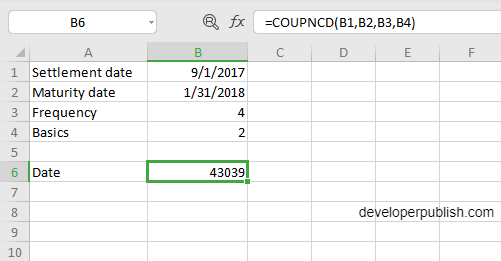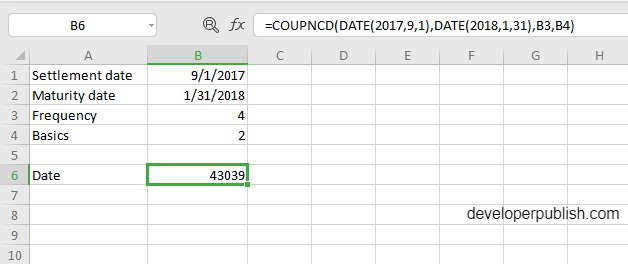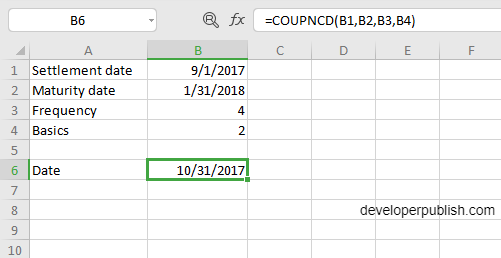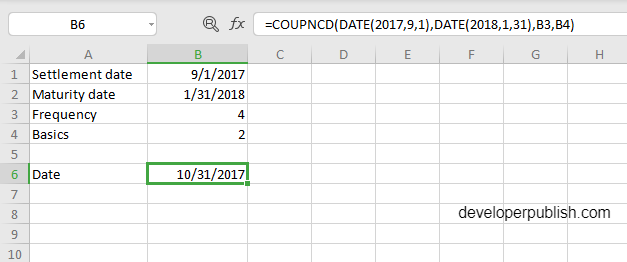# COUPNCD Function in Excel

In this post, you will be guided through simple and easy-to-follow steps on how to use the COUPNCD function in Excel.

Microsoft Office Excel provides the COUPNCD function, which returns the number that represents the next coupon or next date of a coupon after the settlement date.

## COUPNCD Function in Excel

The COUPNCD function helps in calculating the number that represents the next coupon. It returns the next date of the coupon after the settlement date. This is a built-in function under the category Financial Function in Excel.

## COUPNCD Syntax

`=COUPNCD (settlement, maturity, frequency, [basis])`

## The COUPNCD function and arguments

• settlement (Required) – Settlement date of the security.
• maturity (Required) – Maturity date of the security.
• Frequency (Required) – Coupon payments per year (annual = 1, semi-annual = 2, quarterly = 4).
• Basis (Optional) – Day count basis (see below, default =0).

## How to use COUPNCD function in Excel?

• As said in the description, you need the values of all the above arguments to carry out the COUPNCD function to get the correct and desired date of the coupon.
• Enter the arguments in the same order of the syntax, one below the other, as shown in the picture below.
• At this time, in a similar way enter the values of each of the arguments in their corresponding adjacent cells in the worksheet.
• Below the tabulated list of arguments, select a cell and enter “Date”, the cell to the right will display the value of the formula (making identification easier).
• When entering the formula, always start with the “=” operator. After entering the “=” operator enter COUPNCD to initiate the formula followed by an open parenthesis. Excel recognizes “=’ as the start of a formula, if not included, excel will not accept and evade the execution of the function.
• With the parenthesis open, select the first argument value. The position of the cell will be visible in the formula. According to the order of the syntax, the value of the argument must be selected followed by a comma. The change in color of the cells aids to identify the name and of the cells in the formula.
• To conclude, close the parentheses and click enter. The cell which contains the formula will display the date of the coupon. The answer will be displayed in the number format, where we should convert it into the date format.
• Another method to carry out this function, is entering the data in manually in the formula, followed by selecting the values for the last two arguments, where the position of the cell will be visible in the formula. The data should be entered in this format. COUPNCD(DATE(year,month,day),DATE(year,month,day),B3,B4)
• Conclude the formula by giving the closing parenthesis, click enter. The cell which contains the formula will display the date of the coupon. Similarly, the answer will be displayed in the number format, where it should be converted into the date format.

## How to convert number format to date format?

• To convert the number format of the answer to the date format, at first select that particular cell that contains the answer.An example of the first method of syntax insertionAn example of the second method of syntax insertion
• At the top of the page, click on the home tab, in that tab you will find a drop-down box with the format as general.
• Click the arrow, to open the drop-down list, different formats will be displayed, among them click the ‘short date’ format.
• In conclusion, the selected cell which holds the answer will change accordingly (from number format to date format)An example of the first method of syntax insertionAn example of the second method of syntax insertion

.